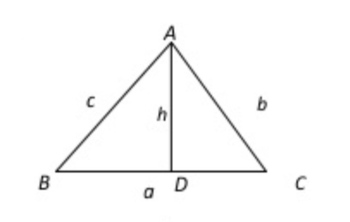# Solutions of triangleSolution of triangle ( introduction and points to be noted)

A triangle has six elements, three angles and three sides. Out of these six, if any three of them are known then the process of determining other remaining three is known as the solution of triangle. The following cases arise while solving a triangle:

1. Three sides are given

2. Three angles are given

3. Two sides and including angle given

4. Two angles and one side given.

5. Two sides and an opposite angle given.

Method of solving Oblique Triangles:

1. If one side and two angles are given, the use Sine Law.

2. If two sides and angle opposite to one of them given, then use Sine Law.

3. If two sides and including an angle are given, then use Cosine Law.

4. If three sides are given, then use Cosine Law.

Note: If there are two solutions while solving a triangle form the given information is called ambiguous.

The possible values of sine may be listed as follows:

1) If sinB> 1, no angle B can be determined, thus, no solution.

2) If sine B= 1, then B =90° therefore, a right angled triangle is determined, thus 1 solution is possible.

3) If sinB<1, then two angles are determined as acute angle B1 and other obtuse as B= 180°- B1. Thus two solutions can be determined. The angles B1 will always be a solution and angle B2 will yield a second solution only if B2 +A < 180°. Otherwise only solution is B1.